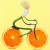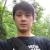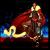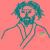## Hello World!

``````\$ newlisp
> _
``````

``````c:\> newlisp
> _
``````

``````> _
``````

``````> (println "Hello World!")
``````

newLISP 打印出输入在 REPL 中提示符后的表达式求值结果，并等待下一次输入.

``````> (println "Hello World!")
Hello World!
"Hello World!"
> _
``````

``````Hello World!
``````

``````"Hello World!"
``````

REPL 会计算任何表达式，不单单是计算函数.

``````> "Hello World!"
"Hello World!"
> _
``````

``````> 1
1
> _
``````

``````println("Hello World!")
``````

``````(println "Hello World!")
``````

``````println("Hello World!")
``````

### 代码和数据是可以互换的

Lisp 的本意是列表处理（List Processor）. Lisp 使用 lists 同时表示代码和数据，它们彼此之间是可以互相转换的。

``````(println "Hello World!")
``````

``````println
``````

``````"Hello World!"
``````

Lisp 总是会将 list 作为函数调用进行执行，除非你引用它，从而表明它只是一个字面形式的符号表达式，也就是 -- 数据。

``````> '(println "Hello World!")
(println "Hello World!")
> _
``````

``````> (eval '(println "Hello World!"))
Hello World!
"Hello World!"
> _
``````

Lisp 程序可以在运行时构建数据的字面量，然后执行它们！

``````> (eval '(eval '(println "Hello World!")))
Hello World!
"Hello World!"
> _
``````

``````> (quote (println "Hello World!"))
(println "Hello World!")
> _
``````

``````> 'x
x
> (quote x)
' x
> '(1 2 three "four")
(1 2 three "four")
> _
``````

``````> 'Hello
Hello
> "Hello"
"Hello"
> _
``````

### 函数的参数

`println` 函数可以拥有任意个数的参数。

``````> (println "Hello" " World!")
Hello World!
" World!"
> _
``````

``````> (quote (println "Hello World!"))
(println "Hello World!")
> _
``````

``````(println "Hello World!")
``````

``````Hello World!
``````

``````> (if true (println "Hello") (println "Goodbye"))
Hello
"Hello"
> _
``````

``````语法: (if condition consequence alternative)

condition（条件）        =>   true
consequence（结果）      =>   (println "Hello")
alternative（替代）      =>   (println "Goodbye")
``````

### 副作用和 Contexts

``````> (set 'hello "Hello")
"Hello"
> (set 'world " World!")
" World!"
> (println hello world)
Hello World!
" World!"
> _
``````

``````> hello
"Hello"
> world
" World!"
> _
``````

newLISP 所有的内置函数是绑定到名字叫 `MAIN` 的 Context.

``````> println
println <409040>
> set
set <4080D0>
> _
``````

``````> 'hello
hello
> _
``````

``````> (eval 'hello)
"Hello"
> _
``````

``````> (eval 'z)
nil
> _
``````

``````> hello
"Hello"
> z
nil
> _
``````

``````> (println hello world)
Hello World!
" World!"
> _
``````

``````> (println 'hello 'world)
?
``````

``````> (println 'hello 'world)
helloworld
world
> _
``````

### 表达式序列

``````> (begin "Hello" " World!")
" World!"
> _
``````

``````> (begin (print "Hello") (println " World!"))
Hello World!
" World!"
> _
``````

``````>[cmd]
(if true
(begin
(print "Hello")
(println " newLISP!"))
(println "So long Java/Python/Ruby!"))[cmd]

Hello newLISP!
" newLISP!"
> _
``````

(注：在提示符后输入多行代码需要在代码开始和结束分别加上 `[cmd]` 直到完成所有代码.）

``````syntax: (if condition consequence alternative)
``````

``````> (exit)
\$
``````

``````> (exit)
c:\>
``````

``````> (exit 3)
``````

``````;  This is a comment

;  hw.lsp

(println "Hello World!")
(exit)
``````

``````\$ newlisp hw.lsp
Hello World!
``````

Windows 上是这样：

``````c:\> newlisp hw.lsp
Hello World!
``````

### 可执行文件和动态链接（Executables and Dynamic Linking）

``````;; uppercase.lsp - Link example
(println (upper-case (main-args 1)))
(exit)
``````

## 在 OSX, Linux 或其他 UNIX 系统：

``````> newlisp -x uppercase.lsp uppercase
> chmod 755 uppercase # give executable permission
``````

## 在 Windows 系统上目标文件需要 `.exe` 后缀

``````\$> newlisp -x uppercase.lsp uppercase.exe
``````

newLISP 会找到环境变量中的 newLISP 可执行文件，并将源文件和它链接在一起.

``````\$> uppercase "convert me to uppercase"
``````

``````CONVERT ME TO UPPERCASE
``````

（Linux 版本的实例暂缺）

``````(import "user32.dll" "MessageBoxA")

(MessageBoxA 0 "Hello World!"
"newLISP Scripting Demo" 0)
``````

``````// echo.c

#include <STDIO.H>
#define DLLEXPORT _declspec(dllexport)

DLLEXPORT void echo(const char * msg)
{
printf(msg);
}
``````

``````(import "echo.dll" "echo")

(echo "Hello newLISP scripting World!")
``````

## 绑定（Binding）

``````(set 'y 'x)
``````

``````(set y 1)
``````

``````> y
x
> x
1
> _
``````

``````(setq y 1)
``````

``````> y
1
> _
``````

``````> (define y 2)
2
> y
2
> _
``````

``````> (set 'x 1 'y 2)
2
> (setq x 3 y 4)
4
> x
3
> y
4
> _
``````

（你应当同我们一起验证这些代码，并记住这些写法）

``````> '((x 1) (y 2))
((x 1) (y 2))
> _
``````

``````> (first '(x 1))
x
> (last '(x 1))
1
> _
``````

``````> (first '((x 1) (y 2)))
(x 1)
> _
``````

``````> (assoc 'x '((x 1) (y 2) (x 3)))
(x 1)
> (lookup 'x '((x 1) (y 2) (x 3)))
1
> _
``````

（函数 `lookup` 还有其它的用途，具体可以查询 newLISP 用户手册.)

### List 是一种递归结构

``````> (first '(1 2 3))
1
> (rest '(1 2 3))
(2 3)
> (last '(1 2 3))
3
``````

``````> (rest '(1))
()
> (rest '())
()
> (first '())
nil
> (last '())
nil
``````

（请注意 newLISP 对列表的定义和 Lisp 和 scheme 其他方言对列表的定义是有区别的。）

``````(define (list-length a-list)
(if (empty? a-list) 0
(+ 1 (list-length (rest a-list)))))
``````

``````(if a-list
...
``````

``````type list ::=  empty-list | first * list
``````

``````> (list-length '(1 2 5))
3
> (length '(1 2 5))
3
> _
``````

``````> (set 'x 1)
1
> (+ x 1)
2
> _
``````

``````> (set 'x 1 'y 2)
2
>
(let  ((x 3) (y 4))
(println x)
(list x y))

3
(3 4)
> x
1
> y
2
> _
``````

`let` 形式和 `begin` 形式很像，除了它在 `let` 块中有一个临时的符号表记录。因为 `let` 中的表达式参数是惰性的，只在 let 的 context 中被展开。如果我们在 `let` 块中查看符号表，它看起来就像下面的关联列表：

``````'((y 4) (x 3) (y 2) (x 1))
``````

``````'((y 2) (x 1))
``````

``````> (begin (+ 1 1) (+ 1 2) (+ 1 3))
4
> (list (+ 1 1) (+ 1 2) (+ 1 3))
(2 3 4)
> (quote (+ 1 1) (+ 1 2) (+ 1 3))
(+ 1 1)
> (quote (2 3 4))
(2 3 4)
> (let () (+ 1 1) (+ 1 2) (+ 1 3))
4
``````

``````> (setq x 3 y 4)
> (let ((x 1) (y 2)) x y)
?
> x
?
> y
?

> (setq x 3 y 4)
> (begin (set 'x 1 'y 2) x y)
?
> x
?
> y
?
``````

``````> (setq x 3 y 4)
> (let ((x 1) (y 2)) x y)
2
> x
3
> y
4

> (setq x 3 y 4)
> (begin (set 'x 1 'y 2) x y)
2
> x
1
> y
2
``````

``````> (setq x 3 y 4)
> (let ((y 2)) (setq x 5 y 6) x y)
?
succeeding> x
?
> y
?
``````

``````> (setq x 3 y 4)
> (let ((y 2)) (setq x 5 y 6) x y)
6
> x
5
> y
4
``````

``````'((y 2) (y 4) (x 3))
``````

``````(setq x 5 y 6)
``````

``````'((y 6) (y 4) (x 5))
``````

``````'((y 4) (x 5))
``````

## 函数（Functions）

``````(define (f x y) (+ x y))
``````

``````(define f (lambda (x y) (+ x y)))

(setq f (lambda (x y) (+ x y)))

(set 'f (lambda (x y) (+ x y)))
``````

`lambda` 表达式定义了一个匿名的函数，或者说是一个没有名字的函数。lambda 表达式的第一个参数是一个形式参数的列表，而接下来的表达式组成了一个惰性的表达式序列，用来描述整个函数的计算过程。

``````> (f 1 2)
3
> ((lambda (x y) (+ x y)) 1 2)
3
> _
``````

``````> (let ((x 1) (y 2)) (+ x y))
3
> ((lambda (x y) (+ x y)) 1 2)
3
> _
``````

lambda 表达式相比 let 表达式唯一的不同就是，它是惰性的，直到传入参数被调用的时候，才会被计算。传入的实际参数会被依次绑定到形式参数的相应符号上，而且有独立的函数作用域。

``````> (setq x 3 y 4)
> ((lambda (y) (setq x 5 y 6) (+ x y)) 1 2)
?
> x
?
> y
?
``````

``````> (setq x 3 y 4)
> ((lambda (y) (setq x 5 y 6) (+ x y)) 1 2)
11
> x
5
> y
4
``````

### 高阶函数

``````> (map eval '((+ 1) (+ 1 2 3) 11))
(1 6 11)
> _
``````

``````> (list (+ 1) (+ 1 2 3) 11)
(1 6 11)
> _
``````

`map` 其实可以做其它很多奇妙的操作：

``````> (map string? '(1 "Hello" 2 " World!"))
(nil true nil true)
> _
``````

``````> (map + '(1 2 3 4) '(4 5 6 7) '(8 9 10 11))
(13 16 19 22)
> _
``````

``````> (+ 1 4 8)
13
> _
``````

``````> (map (fn (x) (= 0 (% x 2))) '(1 2 3 4))
(nil true nil true)
> _
``````

`fn` 是 lambda 的缩写.

``````> (fn (x) (= 0 (% x 2)))
(lambda (x) (= 0 (% x 2)))
> _
``````

``````> (filter (fn (x) (= 0 (% x 2))) '(1 2 3 4))
(2 4)
> _
``````

``````> (index (fn (x) (= 0 (% x 2))) '(1 2 3 4))
(1 3)
> _
``````

``````> (apply + '(1 2 3))
6
> _
``````

``````> (setq op +)
+ <40727D>
> (apply op '(1 2 3))
6
> _
``````

### lambda 列表

``````> (define (f x y) (+ x y z))
(lambda (x y) (+ x y z))
> f
(lambda (x y) (+ x y z))
> _
``````

``````> (first f)
(x y)
> (last f)
(+ x y z)
> _
``````

``````> (setf (nth 1 f) '(+ x y z 1))
(lambda (x y) (+ x y z 1))
> _
``````

(你可以在 newLISP 用户手册中看看函数 `nth-set` 的定义）

``````> (let ((z 2)) (expand f 'z))
(lambda (x y) (+ x y 2 1))
> _
``````

### 动态范围（Dynamic Scope）

``````>
(define f
(let ((x 1) (y 2))
(lambda (z) (list x y z))))

(lambda (z) (list x y z))
> _
``````

``````> f
(lambda (z) (list x y z))
> (setq x 3 y 4 z 5)
5
> (f 1)
(3 4 1)
> (let ((x 5)(y 6)(z 7)) (f 1))
(5 6 1)
``````

Lambda 表达式中的自由变量在调用时动态的从周围的环境中获取，没有从参数传递进来而直接使用的变量就是自由变量。

``````>
(define f
(let ((x 1) (y 2))
(expand (lambda (z) (list x y z)) 'x 'y)))

(lambda (z) (list 1 2 z))
> _
``````

### 函数参数列表

``````>
(define (f z , x y)
(setq x 1 y 2)
(list x y z))

(lambda (z , x y) (setq x 1 y 2) (list x y z))
> _
``````

``````z , x y
``````

``````> (f 3)
(1 2 3)
> _
``````

``````, x y
``````

``````(setq x 1 y 2)
``````

``````>
(define (f z)
(let ((x 1)(y 2))
(list x y z)))

(lambda (z) (let ((x 1)(y 2)) (list x y z)))
> _
``````

``````(define (f z x y)
(if (not x) (setq x 1))
(if (not y) (setq y 2))
(list x y z))
``````

### 宏

``````(define-macro (my-setq _key _value)
(set _key (eval _value)))
``````

``````> (my-setq key 1)
1
> key
1
> _
``````

``````> (my-setq _key 1)
1
> _key
nil
> _
``````

``````(define my-setq
(lambda-macro (_key _value)
(set _key (eval _value))))
``````

``````(define-macro (my-setq )
(eval (cons 'setq (args))))
``````

``````> (cons 1 '(2 3))
(1 2 3)
> _
``````

``````> (my-setq x 10 y 11)
11
> x
10
> y
11
> _
``````

``````'(setq x 10 y 11)
``````

``````(repeat-until condition body ...)
``````

``````(define-macro (repeat-until _condition )
(let ((body (cons 'begin (rest (args)))))
(eval (expand (cons 'begin
(list body
'(while (not _condition) body)))
'body '_condition))))
``````

`repeat-until`:

``````(setq i 0)
(repeat-until (> i 5)
(println i)
(inc i))
; =>
0
1
2
3
4
5
``````

``````(define-macro (repeat-until _condition )
(let ((body (cons 'begin (rest (args)))))
(list (expand (cons 'begin
(list body
'(while _condition body)))
'body '_condition))))
``````

``````> (repeat-until (> i 5) (println i) (inc i))
((begin
(begin
(println i)
(inc i))
(while (> i 5)
(begin
(println i)
(inc i)))))
> _
``````

## Contexts

``````> (context)
MAIN
``````

``````> (setq x 1)
1
> x
1
> MAIN:x
1
> _
``````

``````> (context 'FOO)
FOO
FOO> _
``````

``````FOO> (context? FOO)
true
FOO> (context? MAIN)
true
FOO> (context? z)
nil
FOO> _
``````

``````FOO> (setq x 2)
2
FOO> x
2
FOO> FOO:x
2
FOO> MAIN:x
1
FOO> _
``````

``````FOO> (context MAIN)
> _
``````

``````FOO> (context 'MAIN)
> _
``````

context 不能嵌套 -- 他们都住在一起，之间是平等的。

``````> (setq y 3)
3
> (context FOO)
FOO
FOO> y
nil
FOO> MAIN:y
3
FOO> _
``````

``````FOO> (setq z 4)
4
FOO> (context MAIN)
MAIN
> z
nil
> FOO:z
4
``````

``````> println
println <409040>

> (context FOO)
FOO
FOO> println
println <409040>
``````

``````FOO> (context MAIN)
MAIN
> (setq t 5)
5
> (context 'BAR)
BAR
BAR> t
nil
BAR> (context FOO)
FOO
FOO> t
nil
FOO> (context MAIN)
MAIN
> (global 't)
t
> (context FOO)
FOO
FOO> t
5
FOO> (context BAR)
BAR
BAR> t
5
``````

### 局部作用域（Lexical Scope）

``````> (context 'F)
F
F> (setq x 1 y 2)
2
F> (symbols)
(x y)
F> _
``````

``````F> (define (id z) z )
(lambda (z) z)
F> (symbols)
(id x y z)
F> _
``````

``````F> (context 'B)
B
B> (setq a 1 b 2)
2
B>
``````

``````> (setq x 1 y 2)
2
>
(define foo
(let ((x 3))
(lambda () (list x y))))

(lambda () (list x y))
> (foo)
(1 2)
> _
``````

``````> (let ((x 4)) (foo))
(4 2)
> _
``````

``````> (context 'FOO)
FOO
FOO> (let ((x 5)) (MAIN:foo))
?
``````

``````FOO> (let ((x 5)) (MAIN:foo))
(1 2)
FOO> _
``````

``````FOO> MAIN:foo
(lambda () (list MAIN:x MAIN:y))
FOO> _
``````

``````> foo
(lambda () (list x y))
> _
``````

``````FOO> (let ((MAIN:x 5)) (MAIN:foo))
?
``````

``````FOO> (let ((MAIN:x 5)) (MAIN:foo))
(5 2)
FOO> _
``````

``````(context 'FOO)
(setq r 1 s 2)
``````

``````> (context 'FOO)
FOO>
``````

``````FOO> (setq r 1 s 2)
``````

``````> (begin (context 'FOO) (setq z 5))
FOO> z
nil
FOO> MAIN:z
5
FOO> _
``````

``````(begin (context 'FOO) (setq z 5))
``````

``````(setq MAIN:z 5)
``````

``````(context 'POINT)
(setq x 0 y 0)
(context MAIN)
``````

``````> POINT:x
0
> _
``````

context 同样可以被克隆，因此可以模拟一个简单的类或原型。下面代码中的函数 new，会创建一个名字为 `p` 的新的 context ，如果它不存在的话；同时它会将找到的 context `POINT` 中的符号表合并到这个新的命名空间中。

``````> (new POINT 'p)
p
> p:x
0
> (setq p:x 1)
1
> p:x
1
> POINT:x
0
``````

``````(context 'POINT)
(setq x 0 y 0)
(context MAIN)

(context 'CIRCLE)
(new POINT CIRCLE)
``````

merely (setq radius 1)merely (context MAIN)

``````(context 'RECTANGLE)
(new POINT RECTANGLE)
(setq width 1 height 1)
(context MAIN)
``````

`new` 合并 `POINT` 中的属性 `x``y``CIRCLE` 中，然后在 `CIRCLE` 中建立了另外一个属性 `radius`. `RECTANGLE` 同时也 "继承" 了 `POINT` 所有的属性.

``````(define-macro (def-make _name _ctx )
(let ((ctx (new (eval _ctx) _name))
(kw-args (rest (rest (args)))))
(while kw-args
(let ((slot (pop kw-args))
(val (eval (pop kw-args))))
(set (sym (term slot) ctx) val)))
ctx))
``````

``````(def-make r RECTANGLE x 2 height 2)
``````

``````(define (context->string _ctx)
(let ((str (list (format "#S(%s" (string _ctx)))))
(dotree (slot _ctx)
(push (format " %s:%s" (term slot)
(string (eval (sym (term slot) _ctx))))
str -1))
(push ")" str -1)
(join str)))
``````

``````> (context 'r)
> (setq height 2 width 1 x 2 y 0)
> (context->string 'r)
"#S(r height:2 width:1 x:2 y:0)"
> _
``````

Common Lisp 和 Scheme 都有标记词法作用域的相关函数，这样就可以构建具有函数功能的闭包。newLISP 中的函数同样可以共享一个具有词法作用域的闭包，这就是 context, 这种机制就好像一个对象的方法共享一个类变量一样。到目前为止的实例代码告诉我们 context 也同时可以容纳函数。newLISP 的手册中有好几个事例代码来演示如何用 context 来模拟简单的对象。

（完）

© 著作权归作者所有### 评论(15)#### 引用来自“juqkai”的评论

list-length的代码有错, 应该是:

(define (list-length a-list)
(if (and a-list (first a-list))
(+ 1 (list-length (rest a-list)))
0)
)list-length的代码有错, 应该是:

(define (list-length a-list)
(if (and a-list (first a-list))
(+ 1 (list-length (rest a-list)))
0)
)#### 引用来自“沙枣”的评论#### 引用来自“南湖船老大”的评论#### 引用来自“自主创新”的评论#### 引用来自“tangoboy”的评论

http://lispyscript.com/ lisp版的javascript#### 引用来自“卖姑娘的小火柴”的评论#### 引用来自“哆啦比猫”的评论#### 引用来自“卖姑娘的小火柴”的评论

newLISP你也行 --- newLISP简介

############################################################################# # Name:newLISP你也行 --- newLISP简介 # Author:黄登(winger) # Gtalk:free.winger@gmail.com # Gtalk-Gr......

2012/05/31
1K
0
newLISP 是如何令我惊愕忘形的

2012/07/25
7.6K
7
newLISP 10.7.4 发布，易学易用版类 LISP 语言

newLISP 10.7.4 发布了，此版本与 10.7.x 系列兼容。newLISP 是一个友好的、快速且轻量的类似 Lisp 的脚本语言。它具有 LISP 语言的所有功能，但更容易学习和使用。 此次新增特性包括： 当存...

h4cd
2018/09/16
741
2
newLISP 10.6.3 开发版发布，功能更新和 BUG 修复

newLISP 10.6.3 发布，此版本是开发版本，包括一些新特性和改进： 组件和改进 dolist now also accepts arrays. The net-eval function now returns the evaluation of the last function pa......

oschina
2015/07/13
1K
4
newLISP 10.4.3 发布!修正2个严重Bug!

newLISP 10.4.3 发布了，修复了文件操作 seek 和 close 的严重的问题 下载地址： http://www.newlisp.org/downloads/newLISP-10.4.3-Release.html newLISP是一个类似Lisp语言的、用于一般用途...

wendal
2012/05/08
689
5

EDI 电子数据交换全解指南

EDI（Electronic Data Interchange，电子数据交换)技术使得企业与企业（B2B）实现通信自动化，帮助交易伙伴和组织更快更好地完成更多工作，并消除了人工操作带来的错误。从零售商到制造商、物...

EDI知行软件

3
0
CentOS7的LVM动态扩容

# 问题 CentOS7上面的磁盘空间有点紧张，需要扩容。 解决 查询当前磁盘状态 [root@xxx ~]# lsblkNAME MAJ:MIN RM SIZE RO TYPE MOUNTPOINTfd0 2:0 1 4K ...

3
0
Kafka 0.8 Producer (0.9以前版本适用)

Kafka旧版本producer由scala编写，0.9以后已经废除 示例代码如下： import kafka.producer.KeyedMessage;import kafka.javaapi.producer.Producer;import kafka.producer.ProducerConfig;......

5
0
Giraph源码分析（八）—— 统计每个SuperStep中参与计算的顶点数目

6
0
Navicat 快捷键

13
0